# Bifurcation characteristics of a two stage planetary gear system with varying backlash

Due to the manufacturing accuracy, installation error, lubrication requirement and space reserved for the thermal expansion of gear teeth, the backlash exists objectively. By increasing the backlash B = 30 μ m, the bifurcation diagram and the maximum Lyapunov exponent spectrum of the system are obtained, as shown in Fig. 1 and Fig. 2 respectively.

When the dimensionless excitation frequency Ω is in the interval [1.2, 1.4], the state of the system is more complex. The Lyapunov exponent spectrum of the system appears several intervals jumping from zero to positive. There may be many ways for the system to enter chaos. The Poincare interface, power spectrum and correlation dimension of the system are shown in Fig. 3 ~ Fig. 5 respectively As shown in the figure below, the nonlinear state of the system in this interval is analyzed by Poincare interface, power spectrum and correlation dimension.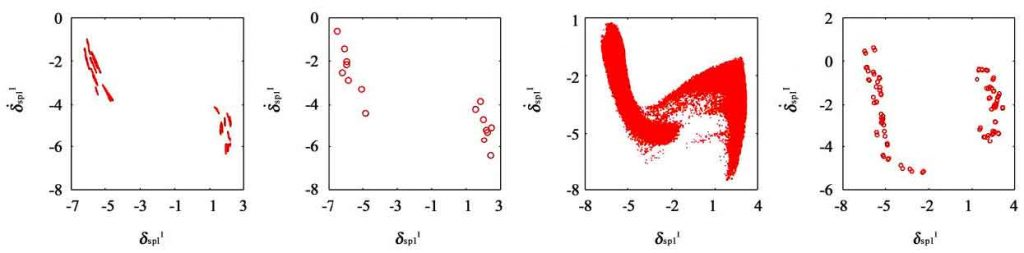(a) 203, quasi periodic motion (b) Ω = 1.214, 16 periodic motion (c) Ω = 1.22, chaotic motion (d) Ω = 1.246, 96 periodic motion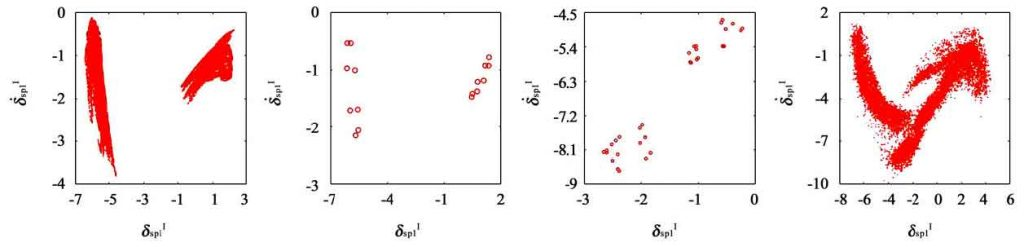(e) 31, chaotic motion (f) Ω = 1.32, 16 periodic motion (g) Ω = 1.324, 32 periodic motion (H) Ω = 1.325, chaotic motion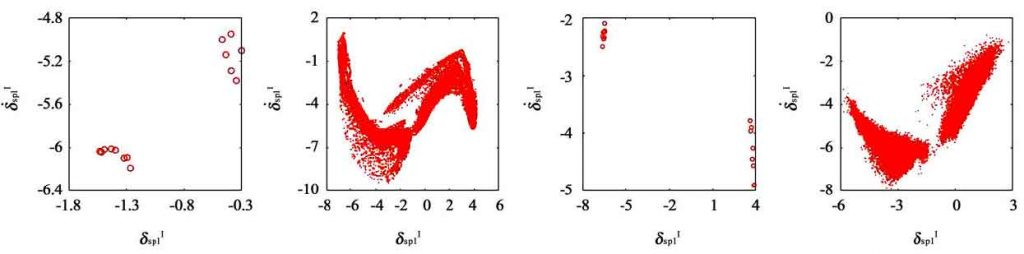(i) 339, 16 periodic motion (J) Ω = 1.35, chaotic motion (k) Ω = 1.378, 16 periodic motion (L) Ω = 1.38, chaotic motion

From Figure 3 to figure 5 It can be seen that with the increase of backlash, the motion between the meshing pairs becomes more complex and chaotic, the chaotic interval of the system increases obviously, the period interval is compressed, the drastic change increases and becomes more intense, and the degree of chaos increases, the dimensionless displacement range of the system increases, the system enters into the chaotic frequency interval ahead of time, and the system experiences quasi period, period, chaos, periodic bifurcation Chaotic process, the vibration of the system becomes more unpredictable.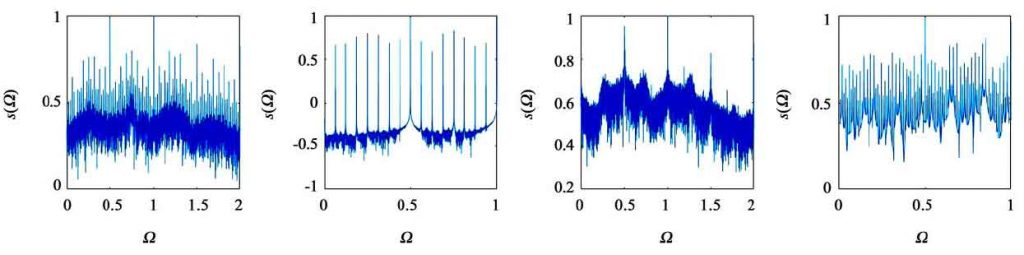(a) 203, quasi periodic motion (b) Ω = 1.214, 16 periodic motion (c) Ω = 1.22, chaotic motion (d) Ω = 1.246, 96 periodic motion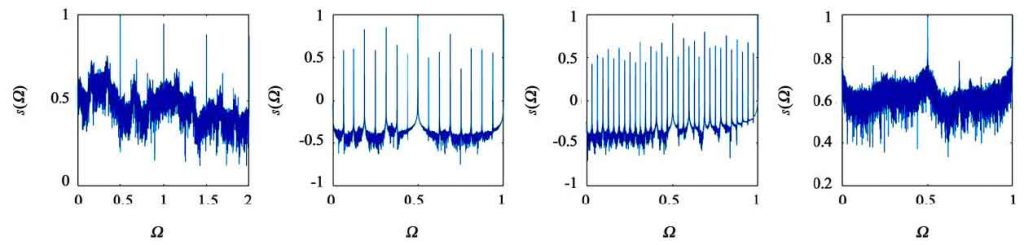(e) 31, chaotic motion (f) Ω = 1.32, 16 periodic motion (g) Ω = 1.324, 32 periodic motion (h) Ω = 1.325, chaotic motion(e) 31, chaotic motion (f) Ω = 1.32, 16 periodic motion (g) Ω = 1.324, 32 periodic motion (h) Ω = 1.325, chaotic motion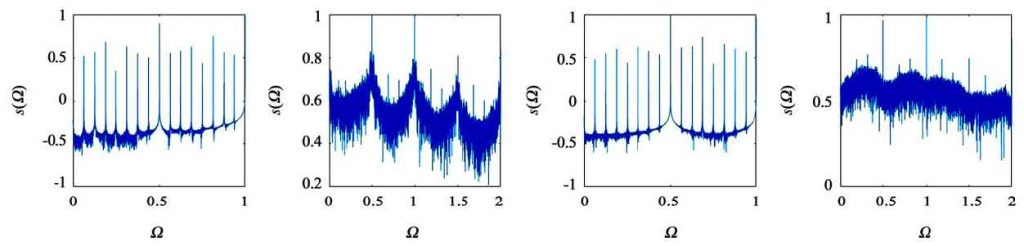(i) 339, 16 periodic motion (J) Ω = 1.35, chaotic motion (k) Ω = 1.378, 16 periodic motion (l) Ω = 1.38, chaotic motion

Compared with the Lyapunov exponent spectrum of the system when the backlash is 20 μ m, it can be found that the Lyapunov exponent spectrum of the system has multiple positive exponents in the chaotic interval, and the value increases significantly, with the largest Lyapunov exponent The exponent determines the divergence rate of phase space orbit, and the motion predictable time can be regarded as the reciprocal of Lyapunov exponent. Therefore, the larger the Lyapunov exponent is, the worse the predictability of motion is, and the more serious the chaos of the system is, which indicates that there is a super chaotic interval in the system, and the chaotic interval of the system increases. In [1.2, 1.4] interval, the motion state of the system Ω is extremely complex. Through the analysis of Poincare cross section and power spectrum, it can be seen that in this interval, the system enters chaos with periodic bifurcation, quasi periodic path and boundary catastrophe It can be seen from the exponential spectrum and bifurcation diagram that before and after the change of backlash, there is a great difference in the exponent corresponding to the shock, and the frequency of the shock occurs in advance, which indicates that the backlash has a great influence on the time and degree of the system from the shock to chaos.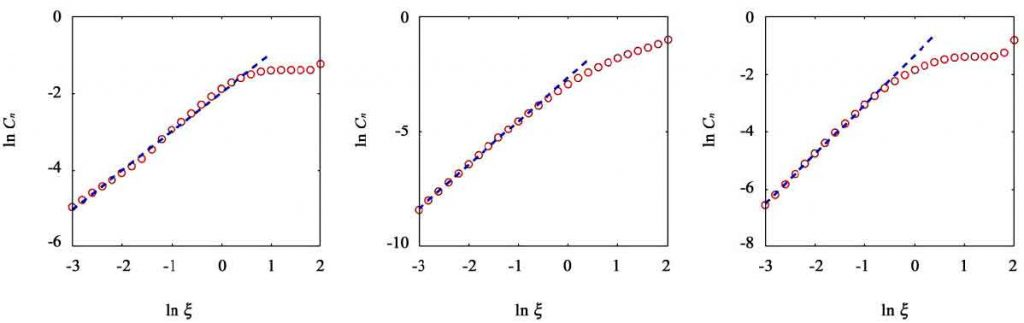(a) 203, quasi periodic motion (b) Ω = 1. 22, chaotic motion (c) Ω = 1. 31, chaotic motion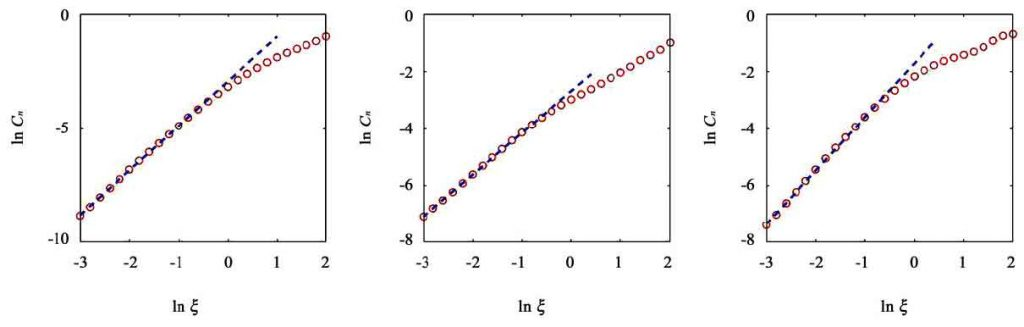(d) 325, chaotic motion (e) Ω = 1. 35, chaotic motion (f) Ω = 1. 38, chaotic motion

When the system is chaotic, there are at most two peaks in the system, which indicates that the system visits at most two different regions of strange attractor, showing periodicity. The correlation dimension of quasi period is 1.01, and the correlation dimension of chaotic state is 1.895, 1.713, 1.946, 1.463, 1.874, respectively. The correlation dimensions are all fractal dimensions. It is verified that chaos appears in the system at this time.